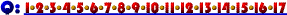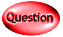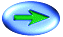1. Davey produces 100 glasses of lemonade with average total cost of 50 cents per glass and average variable cost of 40 cents per glass. What is Davey's total fixed cost?

Since Davey produces 100 glasses of lemonade with average total cost (ATC) of 50 cents per glass we know that his total cost is just ATC x Q or .50 x 100 = \$50.

We also know that his average variable cost (AVC) is 40 cents per glass so his total variable cost is just AVC x Q or .40 x 100 = \$40.

Finally we know that total fixed cost is just total cost - total variable cost or \$50 - \$40 = \$10.Click Here if you don't see topics listed to the left and below.